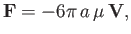Next: Waves in Incompressible Fluids Up: Incompressible Viscous Flow Previous: Axisymmetric Stokes Flow In

# Exercises

1. Consider viscous fluid flow down a plane that is inclined at an angleto the horizontal. Letmeasure distance along the plane (i.e., along the path of steepest decent), and letbe a transverse coordinate such that the surface of the plane corresponds to, and the free surface of the fluid to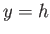. Show that within the fluid (i.e.,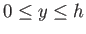)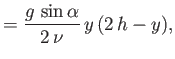where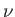is the kinematic viscosity,the density, and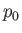is atmospheric pressure.

2. If a viscous fluid flows along a cylindrical pipe of circular cross-section that is inclined at an angleto the horizontal show that the flow rate is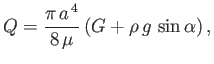where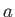is the pipe radius,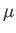the fluid viscosity,the fluid density, andthe pressure gradient.
3. Viscous fluid flows steadily, parallel to the axis, in the annular region between two coaxial cylinders of radiiand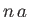, where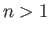. Show that the volume flux of fluid flow is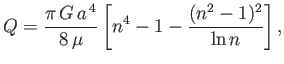whereis the effective pressure gradient, andthe viscosity. Find the mean flow speed.

4. Consider viscous flow along a cylindrical pipe of elliptic cross-section. Suppose that the pipe runs parallel to the-axis, and that its boundary satisfies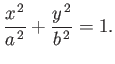Let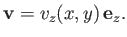Demonstrate that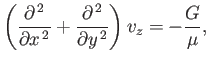whereis the effective pressure gradient, andthe fluid viscosity. Show thatis a solution of this equation that satisfies the no slip condition at the boundary. Demonstrate that the flow rate isFinally, show that a pipe with an elliptic cross-section has lower flow rate than an otherwise similar pipe of circular cross-section that has the same cross-sectional area.

5. Consider a velocity field of the form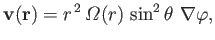where,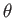,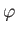are spherical coordinates. Demonstrate that this field satisfies the equations of steady, incompressible, viscous fluid flow (neglecting advective inertia) with uniform pressure (neglecting gravity) provided that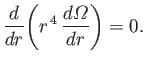Suppose that a solid sphere of radius, centered at the origin, is rotating about the-axis, at the uniform angular velocity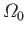, in a viscous fluid, of viscosity, that is stationary at infinity. Demonstrate thatfor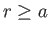. Show that the torque that the sphere exerts on the fluid is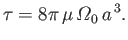6. Consider a solid sphere of radiusmoving through a viscous fluid of viscosityat the fixed velocity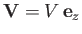. Let,,be spherical coordinates whose origin coincides with the instantaneous location of the sphere's center. Show that, if inertia and gravity are negligible, the fluid velocity, and the radial components of the stress tensor, a long way from the sphere, are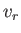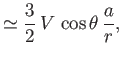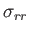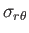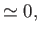respectively. Hence, deduce that the net force exerted on the fluid lying inside a large spherical surface of radius, by the fluid external to the surface, is